Courses

# Competition Level Test: Probability- 1

## 30 Questions MCQ Test Mathematics For JEE | Competition Level Test: Probability- 1

Description
This mock test of Competition Level Test: Probability- 1 for JEE helps you for every JEE entrance exam. This contains 30 Multiple Choice Questions for JEE Competition Level Test: Probability- 1 (mcq) to study with solutions a complete question bank. The solved questions answers in this Competition Level Test: Probability- 1 quiz give you a good mix of easy questions and tough questions. JEE students definitely take this Competition Level Test: Probability- 1 exercise for a better result in the exam. You can find other Competition Level Test: Probability- 1 extra questions, long questions & short questions for JEE on EduRev as well by searching above.
QUESTION: 1

### A coin is tossed n times. The probability of getting head at least once is greater than 0.8. Then the least value of n is

Solution:

Probability of not getting a head = 1/2
Probability of getting at least one head in n tosses is > 0.8 (given)
⇒ 1 -P (getting no head) > 0.8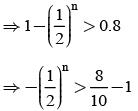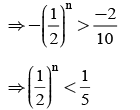Putting values for n, we see that the least value of n satisfying (1) is n=3.

QUESTION: 2

### A and B throw a coin alternately till one of them gets a ‘head’ & wins the game. If A has the first threw, then the probabilities of winning for A and B are respectively.

Solution:

Let S be success &F be failure and let p = probability of getting head = 1/2
q = 1/2
A starts the game then
P (A wins) = P (A wins in first throw) + P (A wins in third throw) + P (A wins in fifth throw) +......... ∞
= P(S) + P(FFS) + P(FFFFS) +......... ∞
= p + q.q.p + q.q.q.q.p +.........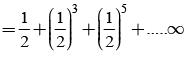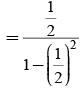This is an infinite GP and hence its sum is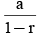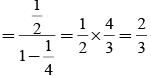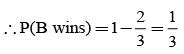QUESTION: 3

### If the probabilities that A&B will die within a year are x & y respectively, then the probability that exactly one of them will be alive at the end of the year is

Solution:

P (A will die within a year) = x
P (B will die within a year) = y
∴ P (Exactly one of them will be alive at the end of the year)
= P (A is alive & B is dead)+ P (A dies B is alive)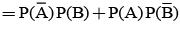(As these are independent events)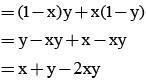QUESTION: 4

Two integers are selected at random from integers 1 to 11. If the sum is even, find the probability that both the numbers are odd.

Solution:

Out of integers from 1 to 11, there are 5 even and 6 odd integers.
Let A – both the numbers chosen are odd
B –sum of the numbers chosen is even.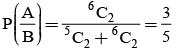QUESTION: 5

A letter is known to have come either from TATANAGAR or from CALCUTTA. On the envelope just two consecutive letters TA are visible. What is the probability that the letters came from TATANAGAR ?

Solution:

Let
X = TATANAGAR
Y = CALCUTTA
P(X) = P(Y) = 1/2
E – Two consecutive letters TA are visible.
Two consecutive letters from X are TA, AT, TA, AN, NA, AG, GA, AR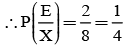Two consecutive letters from Y are CA, AL, LC, CU, UT, TT, TA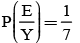∴ By Bayes Theorem,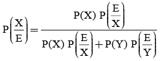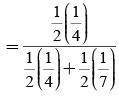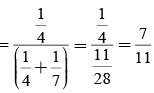QUESTION: 6

There are 5% defective items in a large bulk of items. What is the probability that a sample of 10 items will include not more than one defective item?

Solution:

Let X – No. of defective items in a sample of 10 items drawn successively.
Trial of drawing defective items with replacement are Bernoulli trials.
Probability of success = p = 5/100 = 1/20
∴ Probability of failure =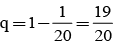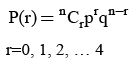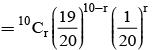(1)
P/not more than 1 defective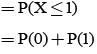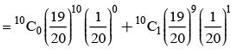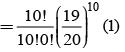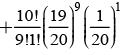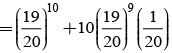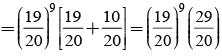QUESTION: 7

Six boys and six girls sit in a row at random. Then the probability that six girls sit together is

Solution:

Let n (s) = total number of ways of seating (permutations) 6 boys and 6 girls
i.e. 12 people = 12P12 = 12!
Let E: six girls sit together.
Then they become one unit
∴ No. of units is 6 boys + 1 unit of girls = 7 units
∴ n (E) = No. of ways in which six girls can sit together is = 7!, 6!
(Here 6! is the number of ways in which the six girls can sit among themselves)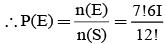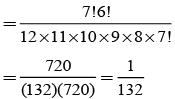QUESTION: 8

The probability for a randomly chosen month to have its 10th day as Sunday is

Solution:

These are seven days in a week and every day can have the chance of being any out of Sunday, Monday, Tuesday, ... Saturday (7 choices)
∴ Reqd. probability = 1/7

QUESTION: 9

A person writes 4 letters and addresses 4 envelopes. If the letters are placed in the envelopes at random, then the probability that all letters are not placed in the right envelope is

Solution:

n(s) = total number of ways of putting 4 letters in 4 envelopes.
= 4P4 = 4! = 24
No. of ways that all letters are placed in right envelope is 1.
∴ n(E)= No. of ways that all letters are not placed in right envelope.
(i.e. at least one letter is put in wrong envelope) = 24 - 1 = 23.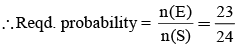QUESTION: 10

A bag contains 5 brown and 4 white socks. A man pulls out 2 socks. The probability that they are of the same colour is:

Solution:

n(S) = Total number of ways of pulling out socks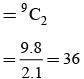Let E be the event that they are of the same colour i.e. either both brown or both white.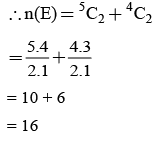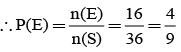QUESTION: 11

From a group of 5 boys and 3 girls, 3 persons are chosen at random. Then the probability that there are more girls than boys is:

Solution:

There are 3 girls and 5 boys
∴ Total number of boys and girls = 8
n(s) = Total number of ways of choosing 3 persons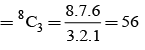Let E be the event that there are more girls than boys.
⇒ (2 girls & 1 boy) or (3 girls & 0 boy)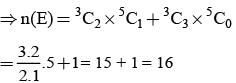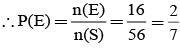QUESTION: 12

Out of 15 tickets marked 1, 2, 3, ... 15, three are drawn at random. The chance that numbers on them are in AP is

Solution:

Remember, if the total number of tickets is (2n + 1) and three (3) tickets are drawn at random; then the number of ways that numbers on the drawn tickets are in AP is n2.
Here 2n + 1 = 15
⇒ 2n = 14
⇒ n = 7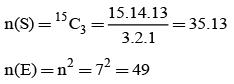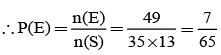QUESTION: 13

Vishnu, Tarun and Amit in order toss a coin. One who throws head first, wins the game. Their respective chances of winning are:

Solution:

Remember that if two people A&B perform an experiment and p is the probability of success in the experiment and q that of failure in the same experiment (and assuming that the game continues indefinitely till one of them meets with success and hence the game); then probability of A & B winning the game are respectively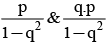If there are 3 people then the probabilities of A, B & C wining the game are respectively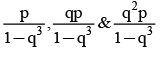∴ we have the respective probabilities of A, B & C winning as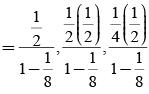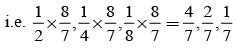QUESTION: 14

Three of the six vertices of a regular hexagon are chosen at random. The probability that the triangle with these vertices is equilateral is:

Solution:

Hexagon has 6 vertices. Three vertices are chosen.
∴ n(S) = Total number of triangles formed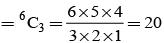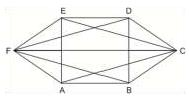Let E : Triangle is equilateral
Out of these 20 triangles, only two triangles ACE and BDF are equilateral and each of their side is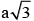where ‘a’ is the side of regular hexagon.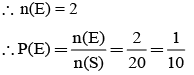QUESTION: 15

A bag contains 50 tickets numbered 1, 2, 3 ... 50, of which five are drawn at random and arranged in ascending order of magnitude (x1< x,< x3< x4< x5), the probability that x3 = 30 is

Solution:

n(S) = Total number of ways of selecting 5 tickets out of 50 tickets = 50C5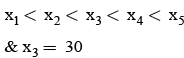∴ The first two tickets are from 29 tickets (numbered 1 to 29) and last two x4& x5 are from next 20 (numbered 31 to 50)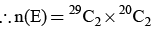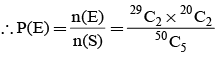QUESTION: 16

In a competition; A, B, C are participating. The probability that A wins is twice that of B and the probability that B wins is twice that of C. The probability that A loses is

Solution:

Let, probability that C wins = p
Then probability that B wins = 2p
Probability of A wins = 2(2p) = 4p
Now
P(A) + P(B) + P(C) = 1
⇒ 4p + 2p + p = 1
⇒ 7p = 1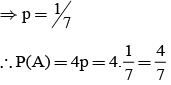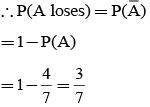QUESTION: 17

A speaks truth in 75% cases and B speaks truth in 80% cases. The probability that they contradict each other in a statement is

Solution:

P(A speaks truth) = 75% = 75/100 = 3/4
∴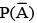= P(A tells a lie)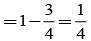P(A speaks truth) = 80% = 80/100 = 4/5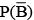= P(B tells a lie)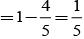∴ P (A & B contradict each other)
= P (A speaks truth & B tells a lie)
+ P (A tells a lie & B speaks truth)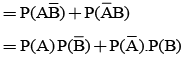(∴ A&B are independent events)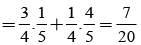QUESTION: 18

A purse contains 4 copper and 3 silver coins; and a second purse contains 6 copper and 2 silver coins. If a coin is taken from one of the two purses, then the probability that it is a copper coin is

Solution: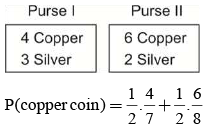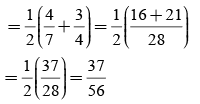QUESTION: 19

A die is thrown three times. If the first throw is a four, find the choice of getting 15 as the sum

Solution:

Let
A – Getting 15 as the sum in a throw of three dice.
B – Getting 4 on the first die.
Required probability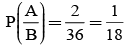(As total number of ways = 1×6×6=36. There are two favourable events (4,6,5) (4,5,6))

QUESTION: 20

Suppose a girl throws a die. If she gets a 5 or 6, she tosses a coin three times and notes the number of heads. If she gets 1, 2, 3 or 4. She losses a coin once and notes whether a head or tail is obtained. If she obtained exactly one head, what is the probability that she threw 1, 2, 3 or 4 with the die?

Solution:

Let  A – Getting exactly one head
E1 – She threw 5 or 6
E2 – She threw 1, 2, 3 or 4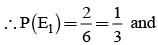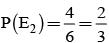Now possible outcomes when throwing a coin three times are
{HHH, HHT, HTH, THH, TTT, TTH, THT, HTT}
Exactly one head = {TTH, THT, HTT}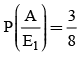When coin is tossed once, the outcomes are {H, T}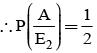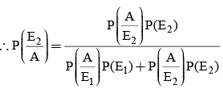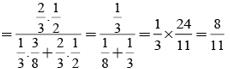QUESTION: 21

Probability that A speaks truth is 4/5. A coin is tossed, a reports that a head appears. The probability that actually there was head is

Solution:

E2 : Tail appears
A : A reports that head appears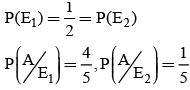∴ Rwquired probability =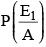By Bayes’ Theorem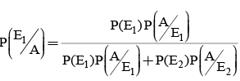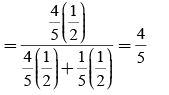QUESTION: 22

Bag I contains 3 red and 4 black balls and bag II contains 4 red and 5 blacks’ balls. One ball is transferred from Bag I to Bag II and then a ball is drawn from Bag II. The ball so drawn is found to be red. Find the probability that the transferred ball is black

Solution:

Let A – Ball drawn from Bag II is red in colour
E1 – Ball transferred from Bag I to Bag II is red
E2 – Ball transferred from Bag I to Bag II is black.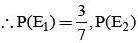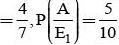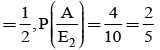By Bayes theorem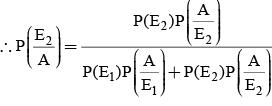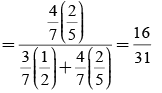QUESTION: 23

A card from a pack of 52 cards is lost. From the remaining cards of the pack, two cards are drawn and are found to be both diamonds. Find the probability that the lost card was a diamond:

Solution:

Let D – Lost card is diamond
D – Lost card is not diamond.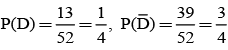When one diamond card is lost, the number of diamond cards are 12 out of 51.
No. of ways of choosing 2 from 12 cards 12C2 .
Similarity, choosing 2 diamond cards out of 51 are 51C2.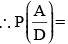probability of getting two cards, when one diamond is lost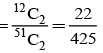When the lost and is not diamond, number of diamond cards are 13 out of 51.
No. of ways of choosing 2 cards when one card is lost which is not diamond =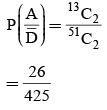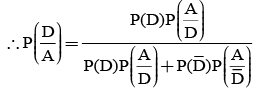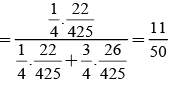QUESTION: 24

In answering a question on a multiple choice test, a student either knows the answer or guesses. Let 3/4 be the probability that he knows the answer and 1/4 be the probability that he guesses. Assuming that a student who guesses at the answer will be correct with probability 1/4. What is the probability that the student knows the answer given that he answered it correctly?

Solution:

Let
A – The answer is correct
E1 – Student. Knows the answer
E2 – Student Guesses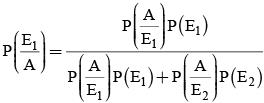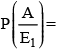We know that he knows and he answers correctly.
= 1.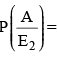We know that he guesses and the answer is correct.
= 1/4
P(E1) = He knows the answer = 3/4
P(E2) = He guesses = 1/4
Substituting all these values in (1) we get :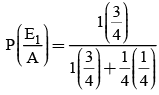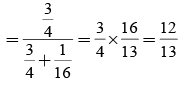QUESTION: 25

On a multiple choice examination with three possible answers for each of the five questions, what is the probability that a candidate would get four or more correct answers just by guessing?

Solution:

Let
X – no. of correct answers by guessing in the set of 5 multiple choice questions. 5 multiple choice questions.
Probability of success = p = 1/3
Probability of failure = q = 1 - p = 2/3
P(r) = nCprqn-r  r = 0,1,2,,....n
P (guessing more than 4 correct answers)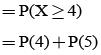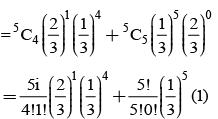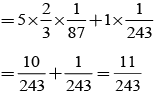QUESTION: 26

Words from the letters of the word PROBABILITY are formed by taking all letters at a time. The probability that both B’s are not together and both I’s are not together is

Solution:
QUESTION: 27

In a n-sided regular polygon, the probability that the two diagonal chosen at random will intersect inside the polygon is

Solution:
QUESTION: 28

A coin is tossed 7 times. Then the probability that at least 4 consecutive heads appear is

Solution:

Let H denote the head,T the tail and * any of the head or tail.
Then,P(H) = 1/2, P(T) = 1/2 and P(∗)=1.
For at least four consecutive heads, we should have any of the following patterns:
Probability
i) HHHH*** (1/2)4×1=1/16
ii)THHHH ** (1/2)5 = 1/32
iii) *THHHH* (1/2)5 = 1/32
iv) **THHHH (1/2)5 = 1/32
Since, all the above cases are mutually exclusive,
the probability of getting at least four consecutive heads (on adding) is 1/16 + 3/32 = 5/32

QUESTION: 29

The probability that a random chosen three-digit number has exactly 3 factor is

Solution:
Sample Space, n(S) = 900
n(E) = 7

P(E) = n(E)/n(S)
= 7/900
QUESTION: 30

Five different games are to be distributed among 4 children randomly. The probability that each child get atleast one game is

Solution:

Total number of ways of distribution is 45
∴ n(S) = 45
Total number of ways of distribution so that each child gets at least one game is
454C1 35 + 4C2 254C3
= 1024 − 4 × 243 + 6 × 32 − 4
∴ n(E)=240
Therefore, the required probability is
n(E)/n(S) = 240/4
= 15/64